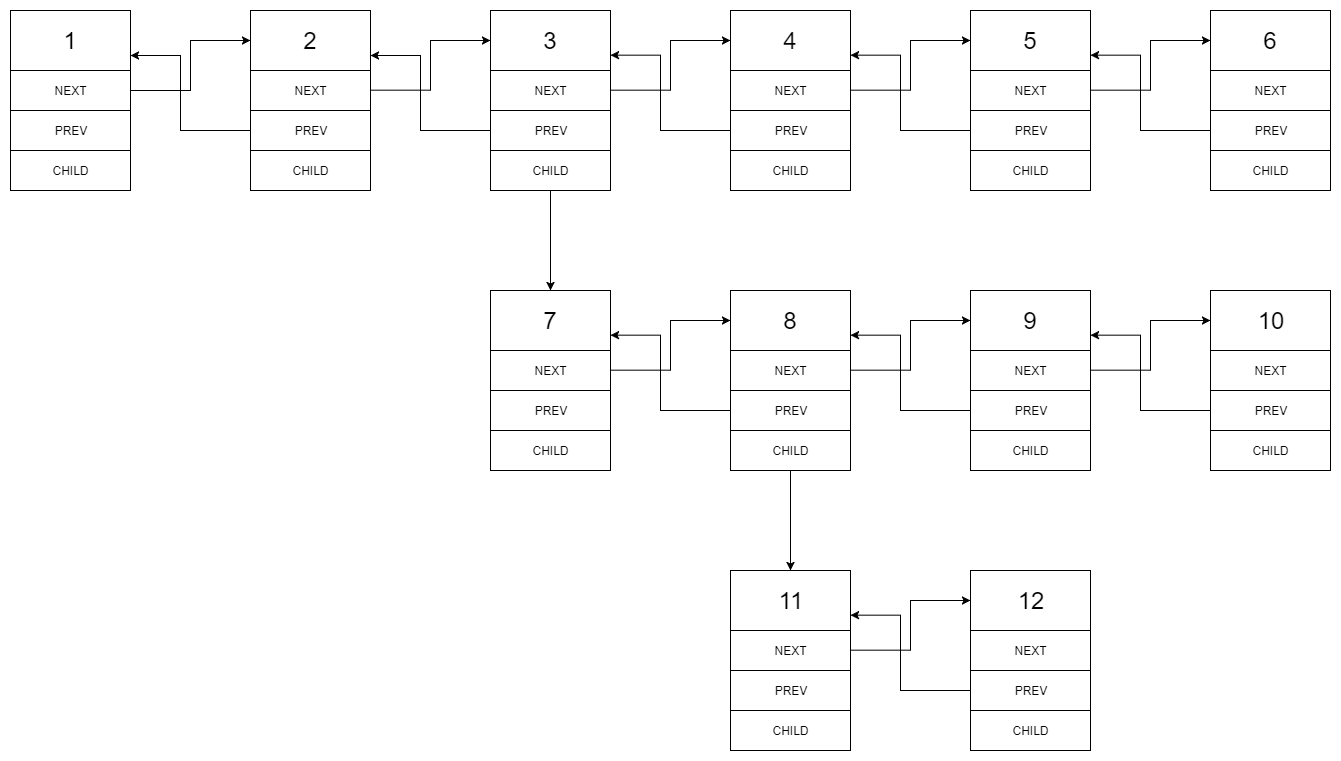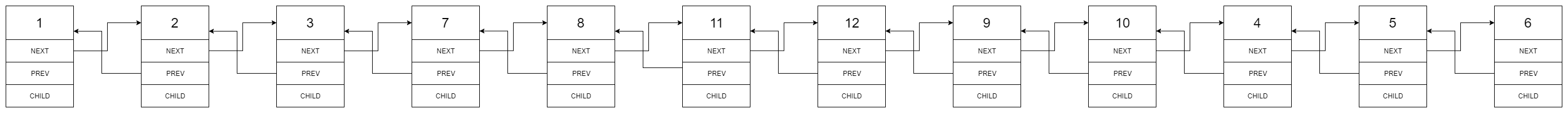##### Welcome to Subscribe On Youtube

Formatted question description: https://leetcode.ca/all/430.html

# 430. Flatten a Multilevel Doubly Linked List (Medium)

You are given a doubly linked list which in addition to the next and previous pointers, it could have a child pointer, which may or may not point to a separate doubly linked list. These child lists may have one or more children of their own, and so on, to produce a multilevel data structure, as shown in the example below.

Flatten the list so that all the nodes appear in a single-level, doubly linked list. You are given the head of the first level of the list.

Example 1:

Input: head = [1,2,3,4,5,6,null,null,null,7,8,9,10,null,null,11,12]
Output: [1,2,3,7,8,11,12,9,10,4,5,6]
Explanation:

The multilevel linked list in the input is as follows:After flattening the multilevel linked list it becomes:Example 2:

Input: head = [1,2,null,3]
Output: [1,3,2]
Explanation:

The input multilevel linked list is as follows:

1---2---NULL
|
3---NULL


Example 3:

Input: head = []
Output: []


How multilevel linked list is represented in test case:

We use the multilevel linked list from Example 1 above:

 1---2---3---4---5---6--NULL
|
7---8---9---10--NULL
|
11--12--NULL

The serialization of each level is as follows:

[1,2,3,4,5,6,null]
[7,8,9,10,null]
[11,12,null]


To serialize all levels together we will add nulls in each level to signify no node connects to the upper node of the previous level. The serialization becomes:

[1,2,3,4,5,6,null]
[null,null,7,8,9,10,null]
[null,11,12,null]


Merging the serialization of each level and removing trailing nulls we obtain:

[1,2,3,4,5,6,null,null,null,7,8,9,10,null,null,11,12]

Constraints:

• Number of Nodes will not exceed 1000.
• 1 <= Node.val <= 10^5

Related Topics:

Similar Questions:

## Solution 1. Recursive

// OJ: https://leetcode.com/problems/flatten-a-multilevel-doubly-linked-list/
// Time: O(N)
// Space: O(H)
class Solution {
public:
Node dummy, *tail = &dummy;
tail->next = node;
node->prev = tail;
tail = node;
if (node->child) {
auto next = flatten(node->child);
tail->next = next;
next->prev = tail;
while (tail->next) tail = tail->next;
}
node->child = NULL;
}
dummy.next->prev = NULL;
return dummy.next;
}
};


## Solution 2. Stack

// OJ: https://leetcode.com/problems/flatten-a-multilevel-doubly-linked-list/
// Time: O(N)
// Space: O(H)
class Solution {
public:
Node dummy, *tail = &dummy;
stack<Node*> s;
while (s.size()) {
auto node = s.top(), child = node->child;
tail->next = node;
node->prev = tail;
tail = node;
node->child = NULL;
if (node->next) s.top() = node->next;
else s.pop();
if (child) s.push(child);
}
dummy.next->prev = NULL;
return dummy.next;
}
};


## Solution 3. Recursive

Return a pair of pointers to first node and last node so that we don’t need to traversel the flattened child again during the recursion.

// OJ: https://leetcode.com/problems/flatten-a-multilevel-doubly-linked-list/
// Time: O(N)
// Space: O(H) where H is the max depth of the child hierarchy.
class Solution {
pair<Node*, Node*> p = {head, NULL}; // first node, last node.
if (next) {
q.second->next = next;
next->prev = q.second;
} else last = q.second;
}
if (!next) p.second = last;
}
return p;
}
public:
}
};


## Solution 4.

// OJ: https://leetcode.com/problems/flatten-a-multilevel-doubly-linked-list/
// Time: O(N)
// Space: O(1)
class Solution {
public:
for (auto p = head; p; p = p->next) {
if (!p->child) continue;
auto next = p->next, q = p->child;
p->next = q;
q->prev = p;
while (q->next) q = q->next;
q->next = next;
if (next) next->prev = q;
p->child = NULL;
}
}
};

• /*
// Definition for a Node.
class Node {
public int val;
public Node prev;
public Node next;
public Node child;
};
*/
class Solution {
Stack<Node> stack = new Stack<Node>();
while (!stack.isEmpty() || temp.next != null || temp.child != null) {
if (temp.next != null) {
if (temp.child != null) {
stack.push(temp);
temp = temp.child;
} else
temp = temp.next;
} else if (temp.child != null) {
Node nextNode = temp.child;
temp.next = nextNode;
nextNode.prev = temp;
temp.child = null;
} else {
Node prevNode = stack.pop();
Node prevNext = prevNode.next;
Node prevChild = prevNode.child;
prevNode.next = prevChild;
prevChild.prev = prevNode;
prevNode.child = null;
temp.next = prevNext;
if (prevNext != null)
prevNext.prev = temp;
}
}
}

}

############

/*
// Definition for a Node.
class Node {
public int val;
public Node prev;
public Node next;
public Node child;
};
*/

class Solution {
return null;
}
Node dummy = new Node();
dummy.next.prev = null;
return dummy.next;
}

private Node preorder(Node pre, Node cur) {
if (cur == null) {
return pre;
}
cur.prev = pre;
pre.next = cur;

Node t = cur.next;
Node tail = preorder(cur, cur.child);
cur.child = null;
return preorder(tail, t);
}
}

• // OJ: https://leetcode.com/problems/flatten-a-multilevel-doubly-linked-list/
// Time: O(N)
// Space: O(H)
class Solution {
public:
Node dummy, *tail = &dummy;
tail->next = node;
node->prev = tail;
tail = node;
if (node->child) {
auto next = flatten(node->child);
tail->next = next;
next->prev = tail;
while (tail->next) tail = tail->next;
}
node->child = NULL;
}
dummy.next->prev = NULL;
return dummy.next;
}
};

• """
# Definition for a Node.
class Node:
def __init__(self, val, prev, next, child):
self.val = val
self.prev = prev
self.next = next
self.child = child
"""

class Solution:
def flatten(self, head: 'Node') -> 'Node':
def preorder(pre, cur):
if cur is None:
return pre
cur.prev = pre
pre.next = cur

t = cur.next
tail = preorder(cur, cur.child)
cur.child = None
return preorder(tail, t)

return None
dummy = Node(0, None, head, None)
dummy.next.prev = None
return dummy.next

############
3
"""
# Definition for a Node.
class Node(object):
def __init__(self, val, prev, next, child):
self.val = val
self.prev = prev
self.next = next
self.child = child
"""
class Solution(object):
"""
:rtype: Node
"""
while node:
node_next = node.next
if node.child:
flattened = self.flatten(node.child)
node.child = None
nextNode = self.appendToList(node, flattened)
node = nextNode
else:
node = node.next

next_node = node.next